This post will serve as a guide on making the above visualization entirely in R (using rayrender), directly from the data source! I wanted to create an example of how your could create a complex, multi-faceted 3D visualization, entirely in R. I've provided all the code so you can generate the visualization from scratch—this can hopefully provide you with several strategies and workflows so you can create beautiful 3D visualizations in R yourself.

We need two sources of data for this visualization: the sea ice extent polygons for both the arctic and antarctic along with the daily sea ice global area. We’ll first start with loading the monthly sea ice extent polygon data from the National Snow and Ice Data Center (NSIDC). NSIDC hosts the data on an FTP server, so we’ll pull the list of files and then download each shapefile individually.

require(RCurl)

ice_dates = c("01_Jan", "02_Feb", "03_Mar", "04_Apr", "05_May", "06_Jun",
"07_Jul", "08_Aug", "09_Sep", "10_Oct", "11_Nov", "12_Dec")

#Arctic

#Load all years of data, for each month
for(i in 1:12) {
sea_url_single = glue::glue("{sea_url}{ice_dates[i]}/")
filenames = getURL(sea_url_single, ftp.use.epsv = FALSE, dirlistonly = TRUE)
filenames = strsplit(filenames, "\n")
filenames = unlist(filenames)
filenames = filenames[!filenames %in% c(".","..")]
for (filename in filenames) {
paste(getwd(), "/", filename,sep = ""))
}
}

And then run the same code, but now for the Antarctic data:

#Antarctic

sea_url_single = glue::glue("{sea_url_south}{ice_dates}/")

#Load all years of data, for each month

for(i in 1:12) {
sea_url_single = glue::glue("{sea_url_south}{ice_dates[i]}/")
filenames = getURL(sea_url_single, ftp.use.epsv = FALSE, dirlistonly = TRUE)
filenames = strsplit(filenames, "\n")
filenames = unlist(filenames)
filenames = filenames[!filenames %in% c(".","..")]
for (filename in filenames) {
paste(getwd(), "/", filename, sep = ""))
}
}

Now, we’re going to load the polygon data into R, and then plot it in 3D over a world map. This requires a few moving parts: the sf package to read in the data, the anglr/silicate/quadmesh packages to project the polygons onto a 3D sphere, and then the rgl package to write the 3D polygon to an OBJ file (that’s then imported by rayrender).

Let’s first load some of our packages:

library(sf)
library(maptools)
library(raster)
library(anglr)
library(silicate)
library(rgl)
library(rayrender)

We’re going to generate two separate visualizations and combine them to create the visualization above. First we’ll generate the polar world map that shows the actual polar ice caps on the right. We’ll render an image for each year and month: when the 3D line graph on the left passes through that month and year, we'll display the corresponding polar polygon data. This way we only have to generate about 360 (12 months * 30 years) images for the right half of the visualization, versus the full 11924 frames for the left.

X

### Love data visualization, mapping, and R? Subscribe to my newsletter!

Our data is given in monthly intervals: let’s write a function that will take the month and year we want and write our two 3D models to the current directory. This function unzips the polygon to a temporary directory, reads the polygon in using sf, generates a Delaunay triangulation (with a maximum triangle size, which preserves interior points and thus captures the curvature of the earth), creates a mesh out of it, projects that mesh onto a sphere, and then writes the mesh to an OBJ file. It does this for both the arctic (arctic.obj) and antarctic (antarctic.obj).

generate_sea_obj = function(yearval, mon_val) {
#South
temp_ice_dir = tempdir()
sea_ice_temp = unzip(sprintf("extent_S_%d%02d_polygon_v3.0.zip",yearval,mon_val),
exdir = temp_ice_dir)

DEL(max_area = 500000000) %>%
as.mesh3d(smooth=TRUE, color="red")
ice_layer_mesh$vb[1:3,] = t(llh2xyz( reproj::reproj(t(ice_layer_mesh$vb[1:3,]),
source = crs(ice_layer),
target="+proj=longlat +datum=WGS84")))
plot3d(ice_layer_mesh,box=FALSE,axes=FALSE)
Sys.sleep(0.2)

rgl::aspect3d("iso")
Sys.sleep(0.2)
rgl::writeOBJ("antarctic.obj")
Sys.sleep(0.2)

rgl::rgl.clear()
#North
sea_ice_temp = unzip(sprintf("extent_N_%d%02d_polygon_v3.0.zip", yearval,mon_val),
exdir = temp_ice_dir)

DEL(max_area = 500000000) %>%
as.mesh3d(smooth=TRUE, color="red")
ice_layer_mesh$vb[1:3,] = t(llh2xyz( reproj::reproj(t(ice_layer_mesh$vb[1:3,]),
source = crs(ice_layer),
target="+proj=longlat +datum=WGS84")))
plot3d(ice_layer_mesh,box=FALSE,axes=FALSE)
Sys.sleep(0.2)

rgl::aspect3d("iso")
Sys.sleep(0.2)

rgl::writeOBJ("arctic.obj")
Sys.sleep(0.2)

rgl::rgl.clear()
}
Figure 1: 3D model of the arctic sea ice.

Now let’s generate the 3D pathtraced polar map on the right. We’ll generate our monthly OBJ file using generate_sea_obj(), place it on top of a sphere with a world map superimposed, load it into a virtual photo studio generated using generate_studio(), and light up the scene with a single sphere light. The data starts in February 1988 and ends in September of 2020, so we’ll skip the first month in 1988 and break out of the loop when it hits October 2020.

yearvals = 1988:2020
mon_vals = 1:12

for(yearval in yearvals) {
for(mon_val in mon_vals) {
if(yearval == 1988 && mon_val == 1) {
next
}
if(yearval == 2020 && mon_val == 10) {
break
}
generate_sea_obj(yearval,mon_val)
generate_studio() %>%
image_texture = "earth.png"))) %>%
image_texture = "earth.png"))) %>%
material=glossy(),angle=c(0,0,0))) %>%
material=glossy(), angle=c(0,180,0))) %>%
render_scene(width=800,height=800,aperture=0, fov=25,sample_method = "stratified",
samples=500, clamp_value=10, lookfrom=c(0,1,10),
filename=glue::glue("seaice_{yearval}_{mon_val}.png"))
}
}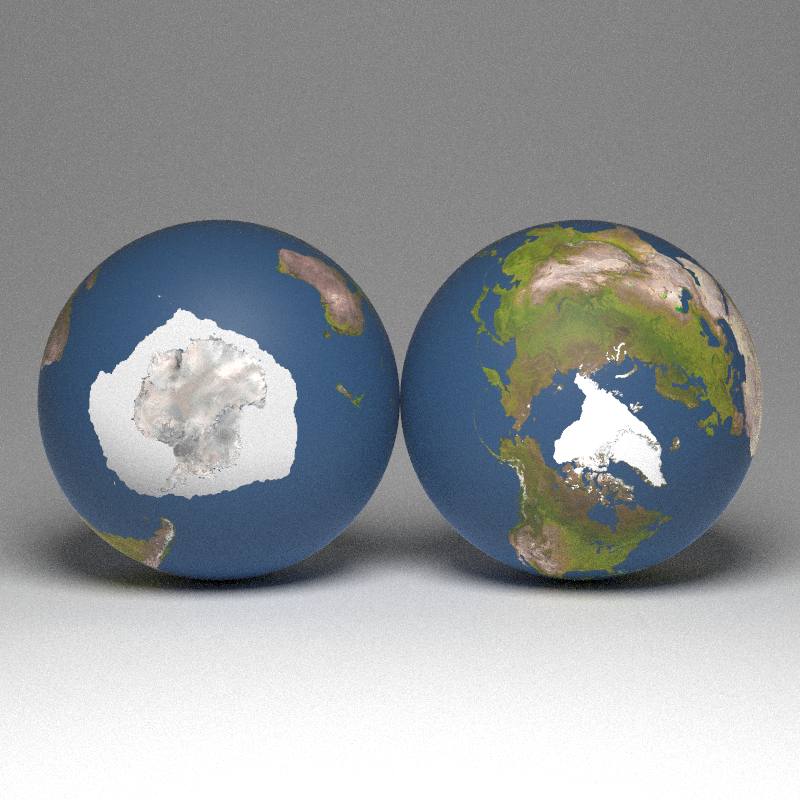Figure 2: Example render for the monthly sea ice polygon data.

Done! Now, let’s generate the line plot on the left. This is a bit more involved 🙂

First, we must load and process the daily sea ice data. I’ve included a cleaned up version of the dataset here. We’ll use dplyr and reshape2 to take clean and transform the data into the format we require to plot it in 3D. Specifically, we need to map the date to a point around a circle. Since we’re working with dates, we’ll take advantage of the great lubridate package.

library(reshape2)
library(dplyr)
library(lubridate)

gt::gt(head(sea_ice))
Month Day X1978 X1979 X1980 X1981 X1982 X1983 X1984 X1985 X1986 X1987 X1988 X1989 X1990 X1991 X1992 X1993 X1994 X1995 X1996 X1997 X1998 X1999 X2000 X2001 X2002 X2003 X2004 X2005 X2006 X2007 X2008 X2009 X2010 X2011 X2012 X2013 X2014 X2015 X2016 X2017 X2018 X2019 X2020
January 1 NA NA 14.200 14.256 NA 14.253 NA NA 14.036 NA NA 14.261 14.319 13.634 14.069 14.035 14.095 14.145 13.804 13.657 14.025 13.823 13.442 13.479 13.590 13.647 13.502 13.160 13.160 13.110 13.206 13.189 13.205 12.896 13.353 12.959 13.011 13.073 12.721 12.643 12.484 12.934 13.102
January 2 NA 14.997 NA NA 14.479 NA 14.103 14.045 NA 14.305 NA 14.313 14.384 13.831 14.092 14.141 14.110 14.258 13.818 13.801 14.097 13.886 13.539 13.385 13.628 13.698 13.538 13.163 13.210 13.207 13.164 13.180 13.232 12.915 13.421 12.961 13.103 13.125 12.806 12.644 12.600 12.992 13.075
January 3 NA NA 14.302 14.456 NA 14.306 NA NA 14.292 NA NA 14.402 14.283 13.847 14.141 14.250 14.042 14.335 13.786 13.837 14.262 13.884 13.630 13.418 13.598 13.876 13.502 13.293 13.267 13.182 13.190 13.267 13.254 12.926 13.379 13.012 13.116 13.112 12.790 12.713 12.634 12.980 13.176
January 4 NA 14.922 NA NA 14.642 NA 14.237 14.240 NA 14.417 NA 14.417 14.321 13.858 14.072 14.255 14.168 14.288 13.791 13.864 14.277 13.913 13.657 13.510 13.623 13.925 13.590 13.313 13.307 13.252 13.275 13.286 13.236 13.051 13.414 13.045 13.219 13.051 12.829 12.954 12.724 13.045 13.187
January 5 NA NA 14.414 14.435 NA 14.494 NA NA 14.489 NA NA 14.381 14.303 13.872 14.185 14.266 14.231 14.304 13.839 14.016 14.217 13.890 13.678 13.566 13.683 14.036 13.617 13.383 13.314 13.361 13.303 13.352 13.337 13.176 13.417 13.065 13.148 13.115 12.874 12.956 12.834 13.147 13.123
January 6 NA 14.929 NA NA 14.880 NA 14.262 14.406 NA 14.515 NA 14.359 14.407 13.958 14.254 14.220 14.295 14.325 13.877 14.139 14.263 14.044 13.806 13.722 13.645 14.075 13.594 13.324 13.265 13.403 13.325 13.447 13.458 13.169 13.404 13.126 13.142 13.138 13.039 12.839 12.772 13.316 13.196
Table 1: Sample of sea ice data, in wide format.

The data is in a wide format—we need it in a long format. Luckily, that’s easy to do with reshape2::melt() (I use 1989 as an example because there are NA values scattered before then):

sea_ice %>%
melt(id.vars=c("Month","Day")) ->
long_sea_ice
gt::gt(head(long_sea_ice[long_sea_ice$variable == "X1989",])) Month Day variable value January 1 X1989 14.261 January 2 X1989 14.313 January 3 X1989 14.402 January 4 X1989 14.417 January 5 X1989 14.381 January 6 X1989 14.359 Table 2: Sample of sea ice data, in long format. We now need to convert the daty of the year to an angle around the circle. We’ll do this by using the yday function in lubridate, which gives the number of days into the year. If you multiply this by $$\frac{1}{365} 2\pi$$, this will give you the angle (in radians) the day corresponds to around the circle. We will also use the built-in dataset month.name in R to easily convert month names to their corresponding numerical values. long_sea_ice %>% mutate(year = substr(variable,2,5)) %>% select(-variable) %>% mutate(datetime = ymd(paste(year,Month,Day))) %>% mutate(day_of_year = yday(datetime), angle_rad = day_of_year/365*2*pi) %>% filter(!is.na(datetime)) %>% filter(datetime > ymd("1988-02-01")) %>% mutate(x_coord = 4*sin(angle_rad), z_coord = 4*cos(angle_rad), y_coord = value) -> data_set_ice ## Warning: 32 failed to parse. data_set_ice$month_vals = match(data_set_ice$Month, month.name) gt::gt(head(data_set_ice)) Month Day value year datetime day_of_year angle_rad x_coord z_coord y_coord month_vals February 2 15.568 1988 1988-02-02 33 0.5680688 2.152021 3.371766 15.568 2 February 3 15.462 1988 1988-02-03 34 0.5852830 2.209741 3.334223 15.462 2 February 4 15.479 1988 1988-02-04 35 0.6024972 2.266807 3.295692 15.479 2 February 5 15.413 1988 1988-02-05 36 0.6197114 2.323201 3.256184 15.413 2 February 6 15.448 1988 1988-02-06 37 0.6369256 2.378907 3.215712 15.448 2 February 7 15.313 1988 1988-02-07 38 0.6541398 2.433907 3.174286 15.313 2 Table 3: Final data frame with circular date positions calculated. We’ll also extract the minimum values from each year to place our annotations: data_set_ice %>% mutate(full_date = glue::glue("{Month} {Day}, {year}")) %>% group_by(year) %>% mutate(min_ext = min(value, na.rm=TRUE)) %>% filter(value == min_ext) -> min_date_values gt::gt(head(min_date_values[,c("full_date","min_ext")])) full_date min_ext September 11, 1988 7.048 September 22, 1989 6.888 September 21, 1990 6.011 September 16, 1991 6.259 September 7, 1992 7.159 September 13, 1993 6.161 Table 4: Sample of annual sea ice minimums. Let’s also extract vectors of the data up until mid-September 2020: pointvals = as.matrix(data_set_ice[1:11294,c("x_coord","y_coord","z_coord")]) year_vals = as.character(data_set_ice[1:11294,"year"]) month_vals = as.numeric(data_set_ice[1:11294,"month_vals"]) day_vals = as.numeric(data_set_ice[1:11294,"day_of_year"]) Now, let’s generate the static portions of our scene. This includes the circular month axis and the vertical sea ice extent axis. #Light intensity intval = 2 #Vertical ticks vert_start = 7 vert_tick_list = list() for(i in seq(0,17)) { vert_tick_list[[i+1]] = segment(start = c(vert_start,i,0), end = c(vert_start+0.2,i,0), radius = 0.01,material=light(intensity = intval,importance_sample = FALSE)) } vert_ticks = do.call(rbind,vert_tick_list) #Vertical labels vert_label_list = list() for(i in seq(0,17)) { vert_label_list[[i+1]] = text3d(label=as.character(i), x = vert_start + 1, y = i, z = 0, text_height = 0.6, angle = c(0,180,0), orientation = "xy", material=light(intensity = intval,importance_sample = FALSE)) } vert_labels = do.call(rbind,vert_label_list) #Horizontal ticks hor_tick_list = list() angles = seq(0,2*pi,length.out = 13)[-13] for(i in seq(1,12)) { hor_tick_list[[i+1]] = segment(start = c(4*sin(angles[i]),0,4*cos(angles[i])), end = c(4.3*sin(angles[i]),0,4.3*cos(angles[i])), radius = 0.01, material=light(intensity = intval,importance_sample = FALSE)) } hor_ticks = do.call(rbind,hor_tick_list) #Horizontal labels months = c("Jan", "Feb", "Mar", "Apr", "May", "Jun", "Jul","Aug", "Sep", "Oct", "Nov", "Dec") month_label_list = list() angles = seq(0,2*pi,length.out = 13)[-13] for(i in seq(1,12)) { month_label_list[[i+1]] = text3d(label=months[i], x = 5.7*sin(angles[j]), y = 0, z = 5.7*cos(angles[j]), text_height = 0.75, orientation = "xy", material=light(intensity = intval,importance_sample = FALSE)) }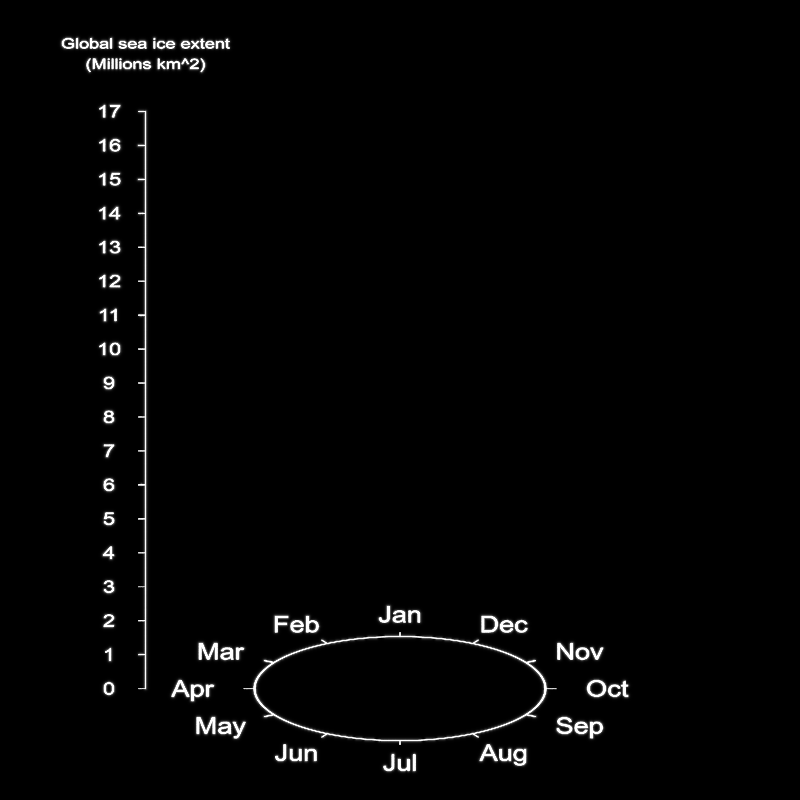Figure 3: Render of static scene elements. We are plotting the path as a series of connected bezier curves, so we need to calculate the control points for each curve (given a series of points we want the curve to travel through). Rayrender has an internal function we can use to do this calculate_control_points(). These are usually calculated by rayrender in the path() function from your desired data points, but this calculation is rather expensive and we don’t want to repeat it every frame, so we’ll pre-compute it and set precomputed_control_points = TRUE in path(). pointlist = rayrender:::calculate_control_points(pointvals) We want to draw two paths: one bright one signifying the last 60 days from the current measurement, and a dim path representing all the measured data points up before then. We can specify this with the parametric u arguments in path(). u_min = seq(0,1,length.out = length(year_vals))-60/length(year_vals) u_min[u_min < 0] = 0 u_max = seq(0,1,length.out = length(year_vals))+1/length(year_vals) u_max[u_max > 1] = 1 In order to label the current value on the axis, we need to extract the y-coordinate in 3D space from the full bezier path. We can do this by finding the single bezier curve that u parametric coordinate falls into, and then calculating the per-curve u and use the bezier curve function to calculate the value at that point. calc_bezier = function(p1,p2,p3,p4, u) { (1-u)^3 * p1 + 3*(1-u)^2 * u * p2 + 3*(1-u) * u^2 * p2 + u^3 * p4 } get_point_along_path = function(pointlist, u) { if(u == 0) { return(pointlist[][1,]) } if(u == 1) { return(pointlist[[length(pointlist)]][4,]) } u_per_point = 1/length(pointlist) u_path = length(pointlist) * u +1 p_index = floor(u_path) pointval = pointlist[[p_index]] u_remain = u_path - p_index calc_bezier(pointval[1,],pointval[2,],pointval[3,],pointval[4,],u=u_remain) } We will then vectorize this function and apply it to every u coordinate along the curve. Using the Vectorize() function is how you show people you’re a professional R superuser who knows how to solve problems with finesse and grace 🙂 point_vec = Vectorize(get_point_along_path, vectorize.args = c("u"), SIMPLIFY = FALSE) points_for_curve = point_vec(pointlist, u=u_max[1:11924,]) Now we render the full thing! Since we aren’t including any non-light materials in this scene, the rendering process happens relatively quickly. This is because the the pathtracing will always terminate at the first ray for each pixel: either it hits a light and terminates, or it doesn’t hit anything and also terminates. Since we have adaptive sampling on, the rendering algorithm quickly hones in on rendering just the elements of our scene. We also include a chunk of code to generate a pulsating year value and minimum area annotations—the year size pulse on January 1st makes the year change more apparent, and the annotation helps drive the narrative of the visualization. for(i in seq(1,length(year_vals),by=1)) { for(j in 1:nrow(min_date_values)) { #Generate annotations for lowest values of current year if(year_vals[i] == min_date_values$year[j] &&
day_vals[i] ==  min_date_values$day_of_year[j]) { annotation_list[[annotation_counter]] = segment(start = c(min_date_values$x_coord[j],
min_date_values$y_coord[j], min_date_values$z_coord[j]),
end = c(min_date_values$x_coord[j]-1.5, min_date_values$y_coord[j],
min_date_values$z_coord[j]), radius = 0.01, material=light(intensity = 1,importance_sample = FALSE)) %>% add_object(text3d(label="Yearly Minimum:", x = min_date_values$x_coord[j]-4,
y = min_date_values$y_coord[j]+0.5, z = min_date_values$z_coord[j],
text_height = 0.5,
angle=c(0,180,0),
orientation = "xy",
material=light(intensity = intval+20,importance_sample = FALSE))) %>%
add_object(text3d(label=min_date_values$full_date[j], x = min_date_values$x_coord[j]-4,
y = min_date_values$y_coord[j], z = min_date_values$z_coord[j],
text_height = 0.5,
angle=c(0,180,0),
orientation = "xy",
material=light(intensity = intval+20,importance_sample = FALSE))) %>%
add_object(text3d(label=glue::glue("{prettyNum(min_date_values$value[j]*1000000, big.mark=',')} km^2"), x = min_date_values$x_coord[j]-4,
y = min_date_values$y_coord[j]-0.5, z = min_date_values$z_coord[j],
text_height = 0.5,
angle=c(0,180,0),
orientation = "xy",
material=light(intensity = intval+20,importance_sample = FALSE)))
#Remove previous year's annotation
if(length(annotation_list) > 1) {
is_cylinder = annotation_list[[annotation_counter - 1]]$shape == "cylinder" annotation_list[[annotation_counter - 1]] = annotation_list[[annotation_counter - 1]][is_cylinder,] annotation_list[[annotation_counter - 1]]$lightintensity = 0.2
}
annotation_counter = annotation_counter + 1
}
}
if(annotation_counter > 1) {
is_text = annotation_list[[annotation_counter-1]]$shape == "xy_rect" intensity_vec = annotation_list[[annotation_counter-1]]$lightintensity[is_text]
if(intensity_vec > intval) {
intensity_vec = intensity_vec - 1
annotation_list[[annotation_counter-1]]\$lightintensity[is_text] = intensity_vec
}
}
all_annotations = do.call(rbind,annotation_list)

path(points = pointlist, width=0.03, type = "flat", u_min = u_min[i], u_max=u_max[i],
precomputed_control_points = TRUE,
importance_sample = FALSE, gradient_type = "rgb",intensity=intval+2,
add_object(path(points = pointlist, width=0.02, type = "flat", u_min = 0, u_max=u_min[i],
precomputed_control_points = TRUE,
importance_sample = FALSE, gradient_type = "rgb",intensity=0.25,
))) %>%
material=light(intensity = intval,importance_sample = FALSE))) %>%
material=light(intensity = intval,importance_sample = FALSE))) %>%
y=points_for_curve[[i]],
z=vert_start * sinpi(camera_angle[counter]/180),
importance_sample = FALSE, gradient_type = "rgb",
intensity=intval+5,
y=0,
z=points_for_curve[[i]],
material=light(color="white",
importance_sample = FALSE,intensity=1))) %>%
end=c(points_for_curve[[i]], points_for_curve[[i]],points_for_curve[[i]]),
material=light(color="white", importance_sample = FALSE,intensity=1))) %>%
x = vert_start ,
y = 19,
z = 0,
text_height = 0.5,
angle = c(0,180,0),
orientation = "xy",
material=light(intensity = intval,importance_sample = FALSE))) %>%
x = vert_start ,
y = 18.4,
z = 0,
text_height = 0.5,
angle = c(0,180,0),
orientation = "xy",
material=light(intensity = intval,importance_sample = FALSE))) %>%
x = 0,
y = 0,
z = 0,
text_height = 1.5 + 1*exp(-day_vals[i]/15),
angle = c(0,180,0),
orientation = "xy",
material=light(intensity = intval,importance_sample = FALSE))) %>%
render_scene(lookfrom=c(30 * sinpi(camera_angle[counter]/180),
20,
-30 * cospi(camera_angle[counter]/180)),
lookat=c(0,8.5,0),
width=800,height=800,fov=0,ortho_dimensions = c(22,22),
min_variance = 0.00001,
samples=100,
filename=glue::glue("seaice{counter}"))
counter = counter + 1
}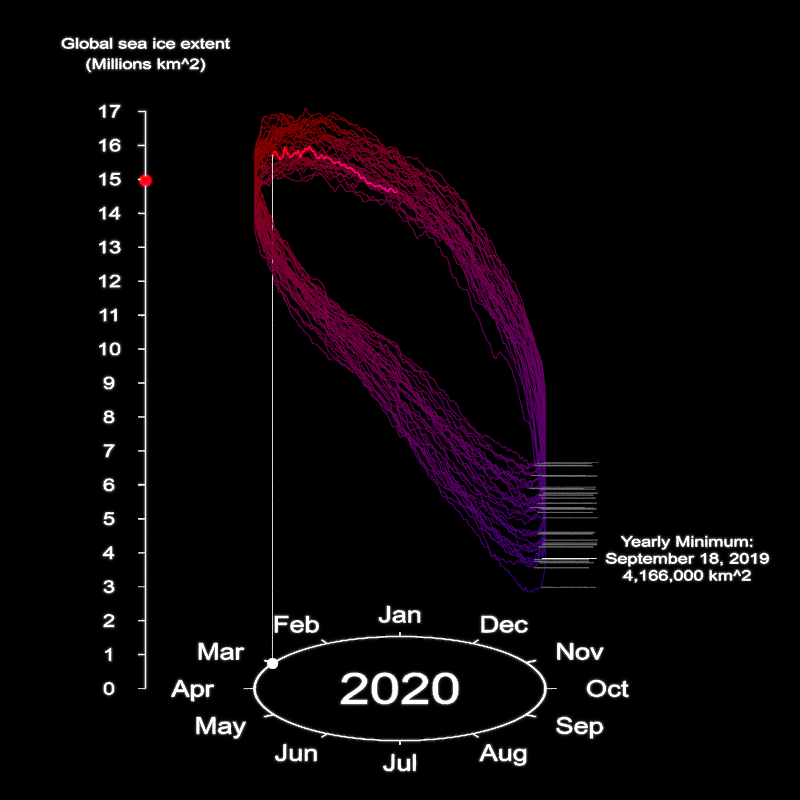Figure 4: Single frame from the render.

Okay, 11924 iterations (and more than a day of rendering!) later, we have all the frames for our visualization! Now let’s add the author information and data source using the magick package. We’ll use the furrr package to easily parallelize the process.

quick_text = function(i) {
title_offset = c(10,5)
title_color = "white"
magick::image_annotate("Data Source: NSIDC",
location = paste0("+", title_offset,"+",title_offset),
size = 16, color = title_color,
font = "sans", gravity = "southeast") %>%
location = paste0("+", title_offset,"+",title_offset),
size = 16, color = title_color,
font = "sans", gravity = "southwest") %>%
magick::image_write(path = glue::glue("seaiceinfo{i}.png"), format = "png")
}
furrr::future_map(1:11924, quick_text)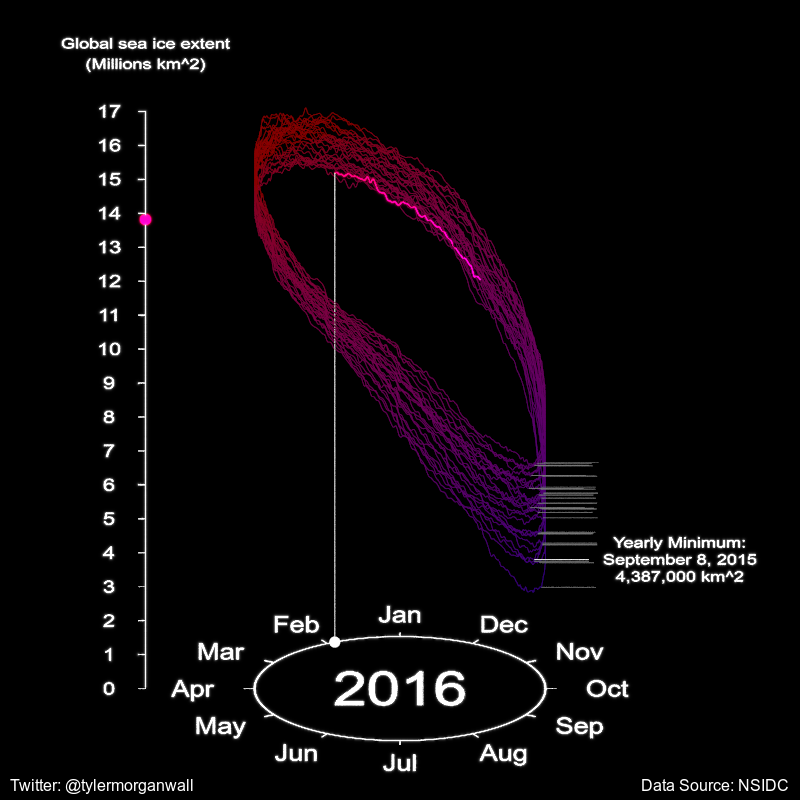Okay, now let’s generate a title. This functionality is built-in to the rayimage package. Our title will span both images, so it should be 1600 pixels wide.

library(rayimage)
title_mat = matrix(0,70,1600)
title_mat %>%
add_title(title_text = "Daily Global Sea Ice Total Area with Monthly Polar Sea Ice Extent, 1988-2020",
title_bar_alpha = 1, title_bar_color = "grey30",
title_color = "white", filename="title")Figure 6: Generated title.

Finally: let’s combine all the images together to form our final visualization using magick! We’ll use furrr again to parallelize the process. This function reads the current value for year_vals and month_vals, and pulls the corresponding render from the world map.

quick_generate_final = function(i) {

magick::image_append(c(line_image,world_image)) %>%
(function(x) magick::image_append(c(title_image,x), stack=TRUE)) %>%
magick::image_write(glue::glue("full_sea_image{i}.png"))
}
furrr::future_map(1:11924,quick_generate_final)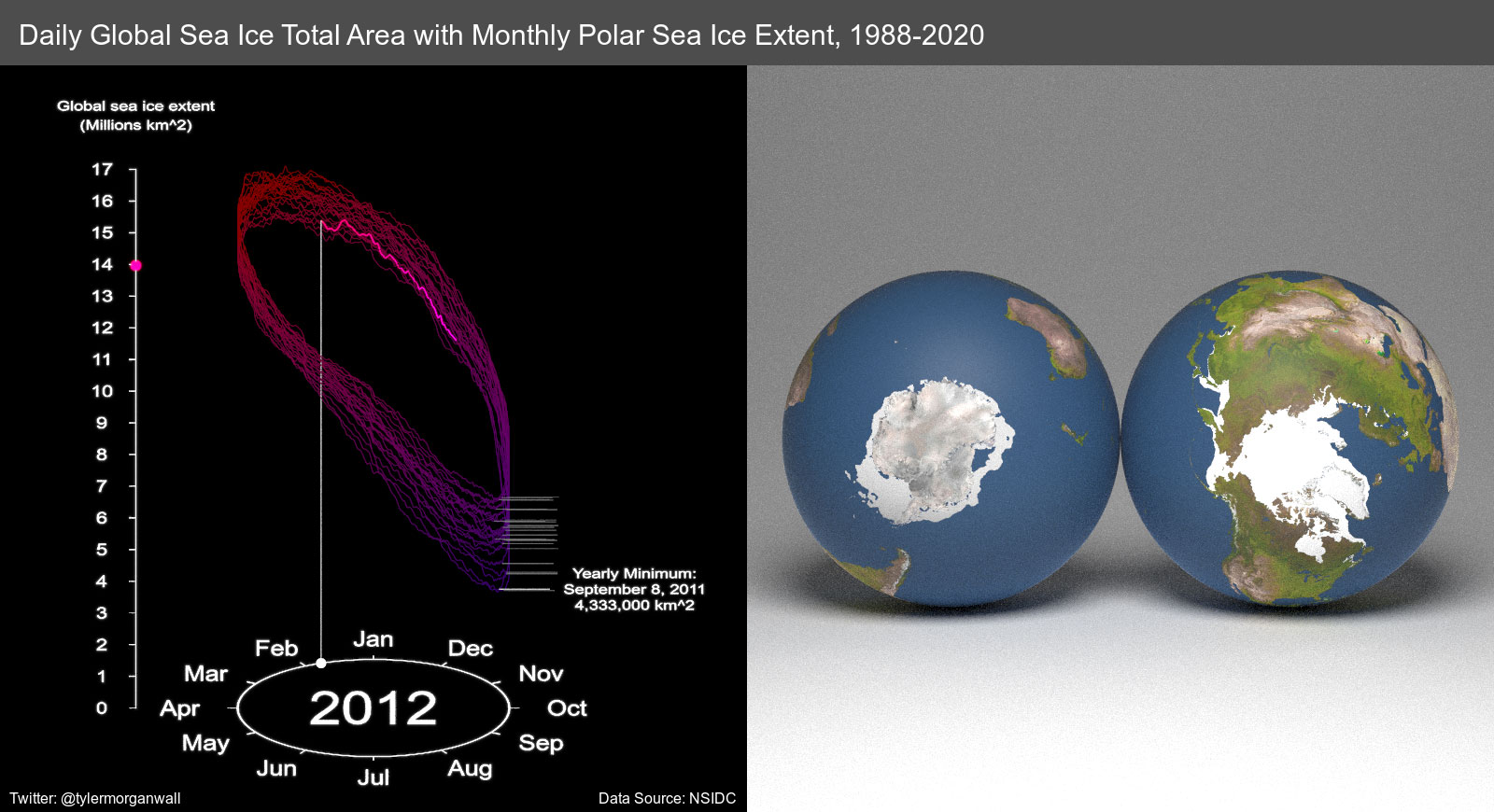Figure 7: Single frame from the full animation.

Let’s also repeat the final frame for a few seconds so the reader can take it in.

for(i in 1:180) {
}
av::av_encode_video(glue::glue("full_sea_image{1:12104}.png"),
output = "full_sea_viz.mp4", framerate = 60)